﻿ 2x Table (two times table) / Division by 2
 HOME ABOUT US SHOP PRIVACY CONTACT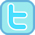# 2x Table (Two times table) / Division by 2

### Free worksheets and resources to help children learn the two times table and the associated division facts.

 1x 2x 3x 4x 5x 6x 7x 8x 9x 10x 11x 12x
 Our Tablet-Friendly 2x Table / Division by 2 Activities 2x Table Practice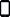2x Table Beat the Clock2x Table Missing Value Practice#### 2x Table (Two Times Table) Printable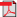Counting in 2s Mad Maths Minutes Sets A & B          Sets C & D          Sets A & B (black)          Sets C & D (black)Recognising Multiples of 2 Mad Maths Minutes Sets A & B          Sets C & D          Sets A & B (black)          Sets C & D (black)2x Table (Visual ) Mad Maths Minutes Sets A & B          Sets C & D2x Table (2 x ? = ) Mad Maths Minutes Sets A & B          Sets C & D          Sets A & B (black)          Sets C & D (black)2x Table (? x 2 = ) Mad Maths Minutes Sets A & B          Sets C & D          Sets A & B (black)          Sets C & D (black)2x Table (mixed orientation) Mad Maths Minutes Sets A & B          Sets C & D          Sets A & B (black)          Sets C & D (black)Division by 2 Pictures Mad Maths Minutes Sets A & B          Sets C & DDivision by 2 Mad Maths Minutes Sets A & B          Sets C & D          Sets A & B (black)          Sets C & D (black)2 x Table / Division by 2 Mad Maths Minutes Sets A & B          Sets C & D          Sets A & B (black)          Sets C & D (black)Counting in 2s2x table fact family arrays2x Table (Coins)#### Interactive Counting in 2s / Multiples of 2 ActivitiesCounting in 2s Mad Maths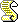Counting in 2s Word Attack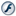Counting in 2s Order OrderMultiples of 2 Interacitve Mad MathsMultiples of 2 Which Category?Multiples of 2 Top of the Class#### Interactive 2x Table (Two Times Table) and 2x Division Facts Activities2x Table Interactive Mad Maths2x Table Pictures Interactive Mad Maths2x Table Shootout (Single Orientation)2x Table Top of the Class2x Table Word Problems2x Table Teacher Invaders2x Table Picture Belt2x Table (single orientation) Word Attack2x Table (mixed orientation) Word Attack2x Table (Repeated Addition to Multiplication)Division by 2 Pictures Interactive2x Table Division Facts Interactive Mad Maths2x Table Division Facts Alien AttackDivision by 2 Word Problems